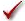## Please enable JavaScript to view this site.

 Analysis checklist: Multiple regression

To check that multiple regression is an appropriate analysis for these data, ask yourself these questions.

###Is the relationship between each X variable and Y linear?

In many experiments, the relationship between X and Y is nonlinear, making multiple regression inappropriate. In some cases you may be able to transform one or more X variables to create a linear relationship. You may also be able to restrict your data to a limited range of X variables, where the relationship is close to linear. Some programs (but none currently from GraphPad Software) can perform nonlinear regression with multiple independent variables.

###Are the residuals (discrepancy between actual and predicted Y values) Gaussian (at least approximately)?

Multiple regression assumes that the distribution of residuals is random and Gaussian.

###Is the variability the same everywhere?

Multiple regression assumes that scatter of data from the predictions of the model has the same standard deviation for all values of X. The assumption is violated if the points with higher (or lower) X values also tend to be further from the best-fit line. The assumption that the standard deviation is the same everywhere is termed homoscedasticity. Prism offers unequal weighting, but then the assumption is that the weighted residuals are, on average, the same everywhere.

###Do you know the X values precisely?

The regression model assumes that all the X values are exactly correct, and that experimental error or biological variability only affects the Y values. This is rarely the case, but it is sufficient to assume that any imprecision in measuring X is very small compared to the variability in Y.

###Are the data points independent?

Whether one value is higher or lower than the regression model predicts should be random, and should not influence whether another point is above or below the line.

###Are you overfitting?

The goal of regression, as in all of statistics, is to analyze data from a sample and make valid inferences about the overall population. That goal cannot always be met using multiple regression techniques. It is too easy to reach conclusions that apply to the fit of the sample data but are not really true in the population. When the study is repeated, the conclusions will not be reproducible.This problem is called overfitting. It happens when you ask more questions than the data can answer— when you have too many independent variables in the model compared to the number of subjects. How many independent variables is too many? For multiple regression, a rule of thumb is to have at least 10–20 subjects (cases; rows in Prism) per independent variable (column in Prism). Fitting a model with five independent variables thus requires about 50 to 100 subjects or cases. That is a rule of thumb, not a strict criterion.

###Are you really overfitting?

If you have fewer cases than variables, your analysis is almost certainly meaningless.